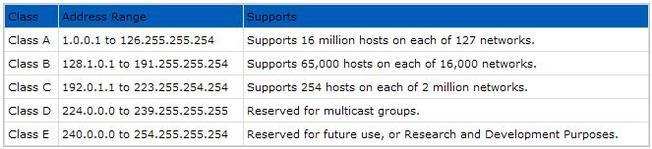## What is Internet Protocol | IPv4 | How to Work

What is Internet Protocol | IPv4 and IPv6 | How to Work Internet Protocol, What is the role in Internet

Short for Internet Protocol, IP is an address of a computer or other network device on a network using IP or TCP/IP. For example, the number "166.70.10.23" is an example of such an address. These addresses are similar to an addresses used on a house and is what allows data to reach the appropriate destination on a network.

There are five classes of available IP ranges: Class A, Class B, Class C, Class D and Class E, while only A, B and C are commonly used. Each class allows for a range of valid IP addresses. Below is a listing of these address. Ranges 127.x.x.x are reserved for loopback or local host, for example, 127.0.0.1 is the common loopback address. Range 255.255.255.255 broadcasts to all hosts on the local network.Every IP address is broke down into four sets of octets that break down into binary to represent the actual IP address. The below chart is an example of the IP 255.255.255.255. If you are new to binary, we highly recommend reading our binary and hexadecimal conversions section to get a better understanding of what we're doing in the below charts.

 IP: Binary value:  Octet value: 25511111111 8

 255 11111111 8 255 25511111111 111111118 8

If we were to break down the IP "166.70.10.23", you would get the below value. The below fist row is the IP address, the second row the binary values, and the third row the binary value calculated to equal the total of that section of the IP address.

 166 10100110 128+32+4+2=166 70 01000110 64+4+2=70

 10 00001010 8+2=10 23 00010111 16+4+2+1=23